: 6 Ground surface : Two dimensional anelastic model : 4 Dust transport

The radiative heating rate is calculated from the radiative flux by using the second order centered scheme.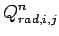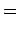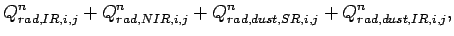(51)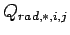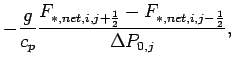(52)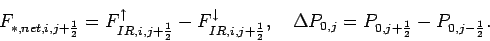The radiative flux is evaluated on the half grid point The subscript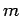shows grid point in the wave number. In the following sections, the superscript which shows time step is omitted.

## 5.1 Radiative transfer of atmospheric CO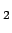The finite difference form of the infrared radiative flux and the Plank function are represented as follows.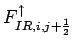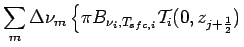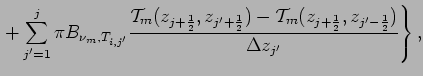(53)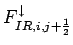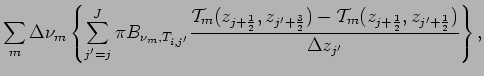(54)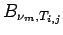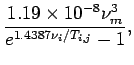(55)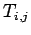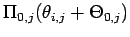(56)

where the averaged transmission function, the equivalent width, and the effective path length are represented as follows.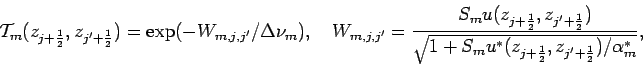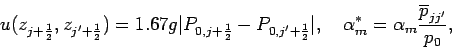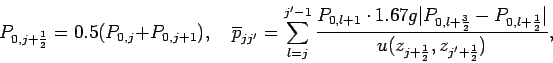The finite form of the near infrared radiative flux and the effective path length are represented as follows.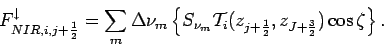(57)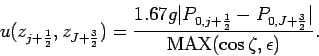where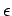is a small parameter to ensure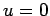when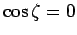.

## 5.2 Radiative transfer of dust

The finite difference form of the solar radiative transfer equation of dust are represented as follows.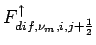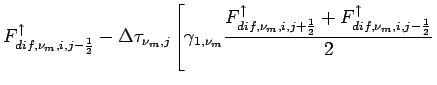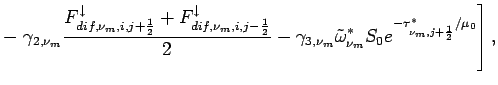(58)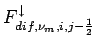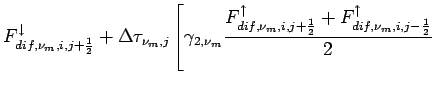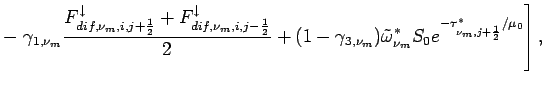(59)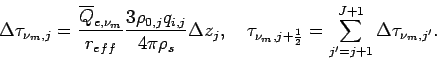(58) and (59) can be represented in matrix form as follows.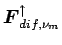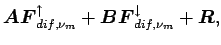(60)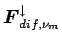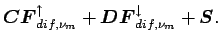(61)

where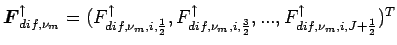and so on. (60) and (61) are solved by iteration method. The elements of the matrixes in (60) and (61) are represented as follows.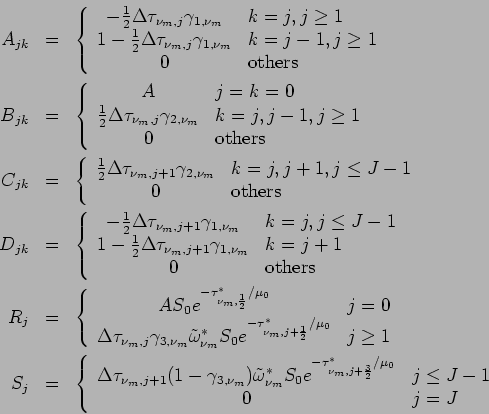The finite difference form of the solar radiative transfer equation of dust are represented as follows.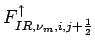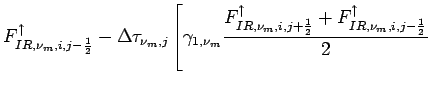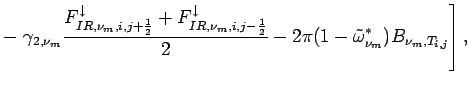(62)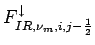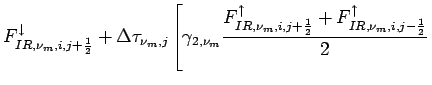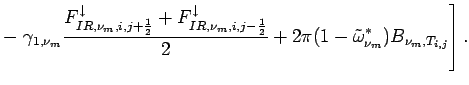(63)

(62) and (63) can be represented in matrix form as follows.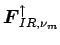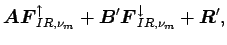(64)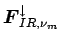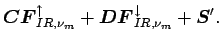(65)

where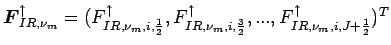and so on. (64) and $B9TNs7A<0$N%@%9%H$N2<8~$-@V30J|64) and $B9TNs7A<0$N%@%9%H$N2<8~$-@V30J|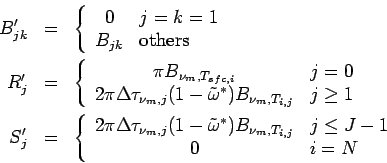: 6 Ground surface : Two dimensional anelastic model : 4 Dust transport
Odaka Masatsugu $BJ?@.(B19$BG/(B4$B7n(B26$BF|(B